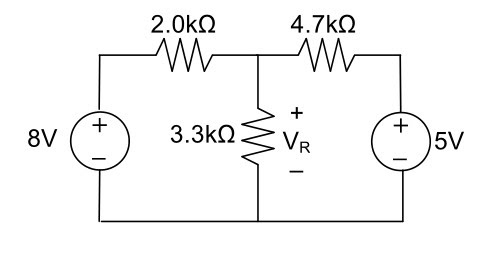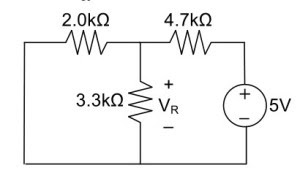# Superposition Theorem with Solved Examples

Hello friends, in this article, we are going to learn a superposition theorem. We will also solve some simple examples using superposition theorem.

## Statement of Superposition Theorem

Superposition theorem states that the response in any element of LTI linear bilateral network containing more than one sources is the sum of the responses produced by the sources each acting independently.

The superposition theorem is not applicable for the power, as power is directly proportional to the square of the current which is not a linear function.

## Steps

1. Select any one source and short all other voltage sources and open all current sources if the internal impedance is not known. If known replace them by their impedance.

2. Find out the current or voltage across the required element, due to the source under consideration.

3. Repeat the above steps for all other sources.

4. Add all the individual effects produced by individual sources to obtain the total current in or across the voltage element.

## Example

Using the superposition theorem, determine the voltage drop and current across the resistor 3.3K as shown in the figure below.## Solution

Step 1: Remove the 8V power supply from the original circuit, such that the new circuit becomes as the following and then measure the voltage across a resistor.Here 3.3K and 2K are in parallel, therefore resultant resistance will be 1.245K.

Using voltage divider rule voltage across 1.245K will be

V1= [1.245/(1.245+4.7)]*5 = 1.047V

Step 2: Remove the 5V power supply from the original circuit such that the new circuit becomes as the following and then measure the voltage across a resistor.Here 3.3K and 4.7K are in parallel, therefore resultant resistance will be 1.938K.

Using voltage divider rule voltage across 1.938K will be

V2= [1.938/(1.938+2)]*8 = 3.9377V

Therefore voltage drop across a 3.3K resistor is V1+V2 = 1.047+3.9377=4.9847

### You may also like

Tags: superposition theorem solved problems, superposition theorem examples, superposition principle.

1.Nice explain...BT please give simple numerical value...so all person can understand easily..thanq

2.Plz tell how to find current in this ex

3.yes plz tell how to cal. current in above problem

4.Let we are given one voltage source and one current source then how can solve the equation.

5.dear priya.... through which resistance do you want to find out the current.

6.if u know about source transformation. Convert the given voltage source into current source parellel with resistance. and u can easily find out the value of current. if ur feeling difficult then u apply kvl or kcl. thank you

7.simple.. first u hv to cosider either current source or voltage source then u will get quation and after solving the equation then u hv to consider again another source except whuch u hv used already then again u will get another equation and then sum of both the equation will be equal to current i

8.How to solve the above circuit if a current source is given?

9.Hloo.. Calculate from above ex fisrt to find the total current in the ckt across 3.3ohms and then solve for the current in 3.3 resistor across

10.Very simple, in last step we got voltage drop across 3.3k ohm has (v1+v2)= 4.98v.
Then current through 3.3k ohm load resistor =[v1+v2]\3.3k ohm .Finally we would get the value current through 3.3k ohm load resistor.

11.nice

12.if i want to find current through resistor which is in series, how can i do that?

13.Please tell me the value of current in this example

14.Use formula of current. I=V/R.
I=4.96/3.3
I=1.50

15.16.1.5mA

17.what do i do when i have four resistors in series and parallel arrangement